Home    |    Teacher    |    Parents    |    Glossary    |    About UsAs you learned in the last lesson, a triangle is the simplest polygon, having three sides and three angles. The sum of the three angles of a triangle is equal to 180 degrees.

Triangles are classified by sides and by angles. Move your cursor over the triangles to learn more.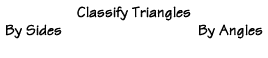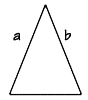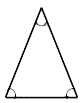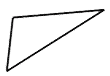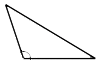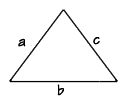Homework Help | Geometry | PolygonsEmail this page to a friendSearch·  Polygon basics·  Triangles·  Quadrilaterals·  Area of polygons     and circles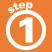First Glance In Depth Examples WorkoutTriangles